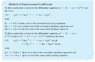# Undetermined coefficients

• rbailey5

## Homework Statement

x(t)^double prime-x(t)=tsint

## The Attempt at a Solution

in this case would I start this as x=t(A(2)+A(1))sint or do i also have to use the cos which makes it
x=t(A(2)+A(1))sint+t(B(2)+B(1))cost?

See attachment.

ehild

#### Attachments

•partikulárismo.JPG
44.6 KB · Views: 420